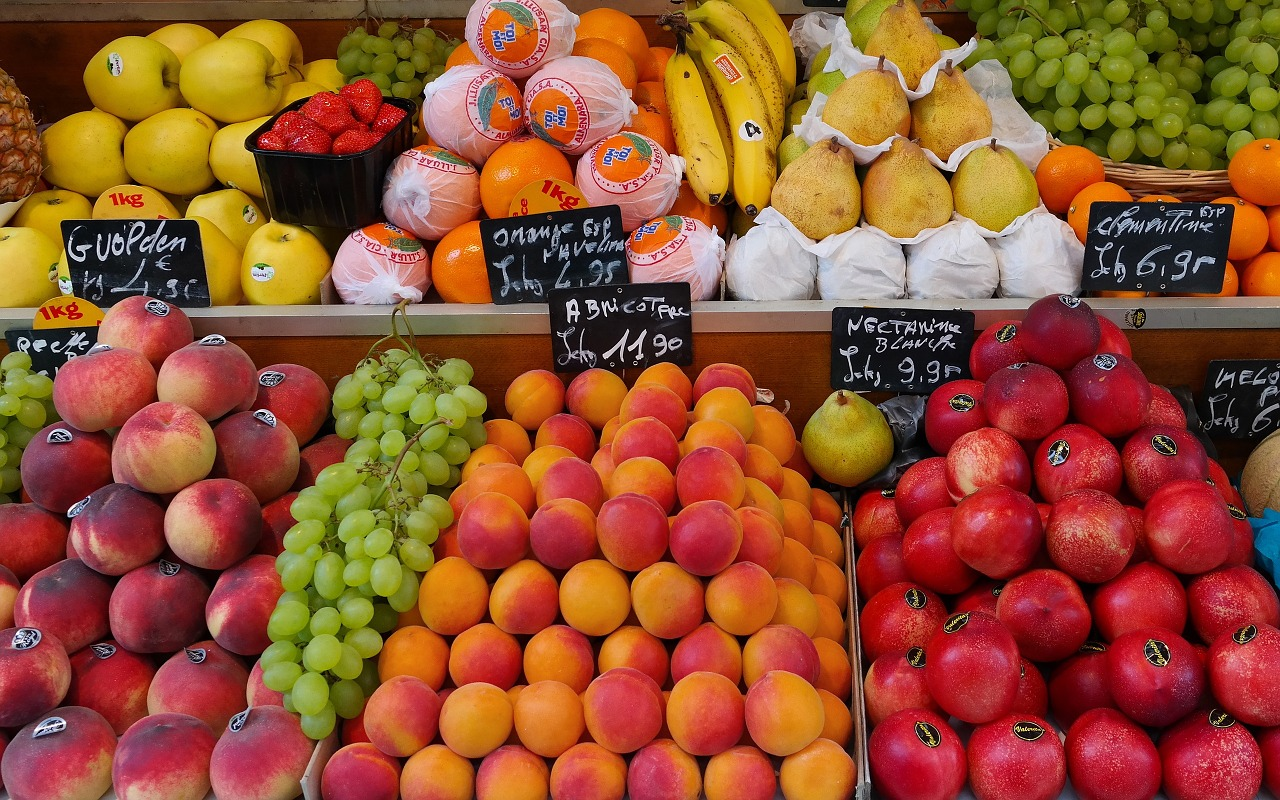# Ways of Thinking and Properties of Operations

Student View (Opens in new window)## Instructor Overview

Students discuss as a class the important ways that listeners contribute to mathematical discussions during Ways of Thinking presentations. Students then use the properties of operations to find the value of each fruit used in equations.

# Key Concepts

Students use the properties of operations to find the value of each fruit used in different equations. By considering several equations, students can match each of the 10 fruits to the whole numbers 0 through 9. This work helps students see why representing unknown numbers with letters is useful.

# Goals and Learning Objectives

• Contribute as listeners during the Ways of Thinking discussion.
• Identify the whole numbers that make an equation true.
• Use the properties of operations, when appropriate, to justify which whole numbers represent unknown values.